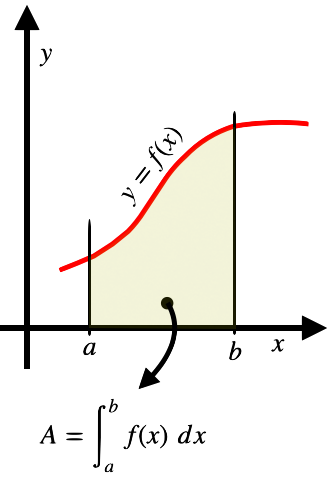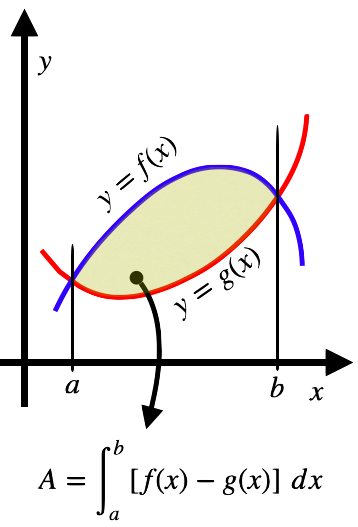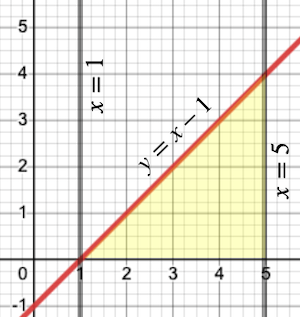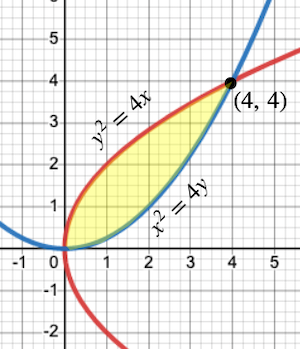# Precalculus - Area and the Definite Integrals

## Introduction

• Integration of a function is simply the opposite of differentiation.
• If $\frac{d}{dx}\left[F\left(x\right)\right]=f\left(x\right)$ then . Here, $F\left(x\right)$ is called the antiderivative (integral) of the function $f\left(x\right)$.
• The integration of a function in which limits of integration are specified is called Definite Integral.
• Mathematically, a definite integral is represented as , where $a$ and $b$ are the lower and upper limits of integration, respectively.
• There are many applications of definite integrals, but most widely, it is used to compute the areas related to plane figures, such as the area between two curves, the area under a curve, etc.

## Fundamental Theorem of Calculus

Theorem 1: If $f\left(x\right)$ be a continuous on the closed interval and $F\left(x\right)$ is defined on the said interval by  then,

${F}^{\text{'}}\left(x\right)=f\left(x\right)$ for any value of $x$, where $x\in \left[a,b\right]$.

Theorem 2: If $f\left(x\right)$ be a continuous function on the closed interval and $F\left(x\right)$ be the antiderivative of $f\left(x\right)$ then,

$=F\left(b\right)-F\left(a\right)$

## Properties of Definite Integrals

• , where $a.
• , where $c$ is any constant.
• If $f\left(x\right)\ge 0$ on , then
• If $f\left(x\right)\le 0$ on , then
• If $f\left(x\right)\ge g\left(x\right)$ on , then
• if $f\left(-x\right)=f\left(x\right)$ i.e. $f\left(x\right)$ is an even function
• if $f\left(-x\right)=-f\left(x\right)$ i.e. $f\left(x\right)$ is an odd function

## Evaluation of Definite Integrals by Substitution

• For evaluating integrals, sometimes, we change the variable of integration to some other variable in order to reduce the given integral to one of the fundamental integrals. This method is called the evaluation of integrals by the method of substitution.
• Let us understand the concept of integration by substitution method by an example.

Evaluate .

Let ${x}^{7}+1=t$, then

Therefore,

$=\frac{2}{3}{t}^{3}{2}}$

$=\frac{2}{3}{\left({x}^{7}+1\right)}^{3}{2}}$

Hence, $=\frac{2}{3}{\left[{\left({x}^{7}+1\right)}^{3}{2}}\right]}_{-1}^{1}$

$=\frac{2}{3}\left[{\left({\left(1\right)}^{7}+1\right)}^{3}{2}}-{\left({\left(-1\right)}^{7}+1\right)}^{3}{2}}\right]$

$=\frac{2}{3}\left[{\left(1+1\right)}^{3}{2}}-{\left(-1+1\right)}^{3}{2}}\right]$

$=\frac{2}{3}\left[{2}^{3}{2}}-0\right]$

$=\frac{2}{3}×2\sqrt{2}$

$=\frac{4\sqrt{2}}{3}$

## Computing Areas by Definite Integral

The definite integral is widely used to compute areas related to plane figures such as the area under a curve and the area between two intersecting curves.

Computing Area under a Curve:

• The area bounded by the function $f\left(x\right)$, the x-axis, and the two ordinates $x=a$ and $x=b$, is given by  as shown in the figure.Computing Area between two intersecting Curves:

• The area bounded by the two intersecting curves $y=f\left(x\right)$ and $y=g\left(x\right)$ and the ordinates $x=a$ and $x=b$, is given by , where $f\left(x\right)\ge g\left(x\right)$.## Solved Examples

Example 1. Evaluate the definite integral .

Solution:

$=5{\left[\frac{{x}^{4+1}}{4+1}\right]}_{2}^{3}-6{\left[\frac{{x}^{2+1}}{2+1}\right]}_{2}^{3}-2{\left[x\right]}_{2}^{3}$

$=5{\left[\frac{{x}^{5}}{5}\right]}_{2}^{3}-6{\left[\frac{{x}^{3}}{3}\right]}_{2}^{3}-2{\left[x\right]}_{2}^{3}$

$={\left[{x}^{5}\right]}_{2}^{3}-2{\left[{x}^{3}\right]}_{2}^{3}-2{\left[x\right]}_{2}^{3}$

$=\left[{3}^{5}-{2}^{5}\right]-2\left[{3}^{3}-{2}^{3}\right]-2\left[3-2\right]$

$=\left(243-32\right)-2×\left(27-8\right)-2×\left(1\right)$

$=211-38-2$

$=171$

Example 2. Evaluate .

Solution: Let ${x}^{9}+1=t$, then

Therefore,

$=\frac{2}{3}{t}^{3}{2}}$

$=\frac{2}{3}{\left({x}^{9}+1\right)}^{3}{2}}$

Hence, $=\frac{2}{3}{\left[{\left({x}^{9}+1\right)}^{3}{2}}\right]}_{0}^{1}$

$=\frac{2}{3}\left[{\left({\left(1\right)}^{9}+1\right)}^{3}{2}}-{\left(0+1\right)}^{3}{2}}\right]$

$=\frac{2}{3}\left[{\left(1+1\right)}^{3}{2}}-{\left(1\right)}^{3}{2}}\right]$

$=\frac{2}{3}\left[{2}^{3}{2}}-1\right]$

$=\frac{2}{3}×\left(2\sqrt{2}-1\right)$

$=\frac{4\sqrt{2}-2}{3}$

Example 3: Find the area bounded by the curve $y=x-1$, the x-axis, $x=1$, and $x=5$.

Solution: The bounded region is shown in the figure below.Because $y=f\left(x\right)\ge 0$ on , the area of the bounded region is

$={\left[\frac{{x}^{2}}{2}-x\right]}_{1}^{5}$

$=\left[\frac{{5}^{2}}{2}-5\right]-\left[\frac{{1}^{2}}{2}-1\right]$

$=\left[\frac{25}{2}-5\right]-\left[\frac{1}{2}-1\right]$

$=\frac{15}{2}+\frac{1}{2}$

$=\frac{16}{2}$

Example 4: Find the area bounded by the curve ${y}^{2}=4x$ and ${x}^{2}=4y$.

Solution:  and

To get the common points on both curves, we put $y=\frac{{x}^{2}}{4}$ in the equation $\left(i\right)$:

${\left(\frac{{x}^{2}}{4}\right)}^{2}=4x$

$\frac{{x}^{4}}{16}=4x$

${x}^{4}=64x$

${x}^{4}-64x=0$

$x\left({x}^{3}-64\right)=0$

$x\left({x}^{3}-{4}^{3}\right)=0$

$x\left(x-4\right)\left({x}^{2}+4x+16\right)=0$

$x=0$$x=4$

So, the common points are  and .

Because $\sqrt{4x}\ge \frac{{x}^{2}}{4}$ on  as shown in the figure, the area of the bounded region is$={\left[\frac{2{x}^{\frac{3}{2}}}{\frac{3}{2}}-\frac{1}{4}\frac{{x}^{3}}{3}\right]}_{0}^{4}$

$={\left[\frac{4{x}^{\frac{3}{2}}}{3}-\frac{{x}^{3}}{12}\right]}_{0}^{4}$

$=\left[\frac{4×{\left(4\right)}^{\frac{3}{2}}}{3}-\frac{{\left(4\right)}^{3}}{12}-0\right]$

$=\left[\frac{32}{3}-\frac{16}{3}\right]$

## Cheat Sheet

• Integration is simply the opposite of differentiation.
• Definite integrals always have well-defined limits of integration.
• Some of the properties of the definite integral are mentioned below.
• , where $a.
• , where $c$ is any constant.
• If $f\left(x\right)\ge 0$ on , then
• If $f\left(x\right)\le 0$ on , then
• If $f\left(x\right)\ge g\left(x\right)$ on , then
• if $f\left(x\right)$ is an even function else zero.
• In the method of Integration by substitution, we change the variable of integration to some other variable in order to reduce the given integral to one of the fundamental integrals.
• The area bounded by the function $f\left(x\right)$, the x-axis, and the two ordinates $x=a$ and $x=b$, is given by .
• The area bounded by the two intersecting curves $y=f\left(x\right)$ and $y=g\left(x\right)$ and the ordinates $x=a$ and $x=b$, is given by , where $f\left(x\right)\ge g\left(x\right)$.

## Blunder Areas

• The area of a region is never negative. If a function $f\left(x\right)\le 0$ on the closed interval , then the area of the region between the function, the x-axis, and the ordinates $x=a$ and $x=b$ is

(since the area lies below the x-axis).

• If a function is continuous in an interval, it is also integrable in this given interval.
• The derivative of an antiderivative is the original function.
• The skill of finding the antiderivative of different functions is a prerequisite in evaluating definite integrals.
• The areas of regions bounded by curves at a certain interval are just approximations of the exact area.